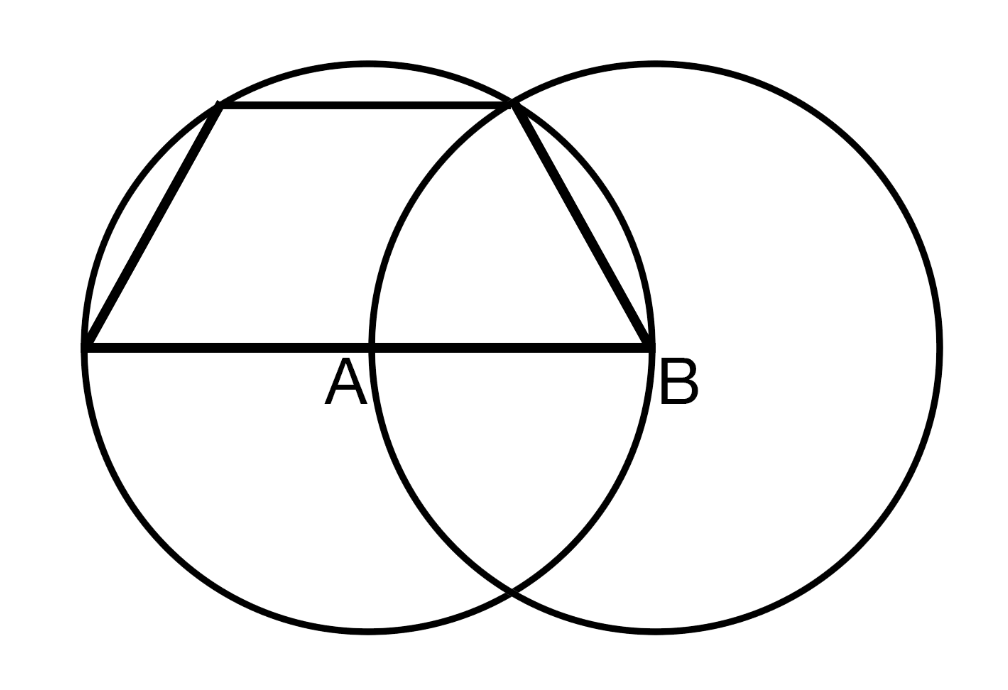# The trapezoid is trapped in the circle!

Geometry Level 2We see circles A and B of radius 1 in the diagram. Find the area of the trapezoid. If this area is expressible in the form $\dfrac{a \sqrt{b}}{c}$, where $a, c$ are coprime and $b$ is square-free, find $a + b + c$.

×